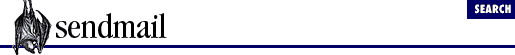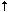home | O'Reilly's CD bookshelfs | FreeBSD | Linux | Cisco | Cisco Exam

#Chapter 33Database Macros## 33.4 Use Maps with \$( and \$) in Rules

The information in database files is accessed for use in the RHS of rules. This is the syntax:

```\$( ```

name key
```
\$)```

The ``` key ``` is looked up in the database whose symbolic name (as declared with the ``` K``` configuration command) is ``` name ``` (see Section 33.3 ) If the ``` key ``` is found, the entire expression, including the ``` \$(``` and ``` \$)``` , is replaced with the value from the database entry for that ``` key ``` . Any ``` suffix ``` , as specified with the ``` -a``` switch (see Section 33.3.4.2 ) in the ``` K``` configuration declaration for ``` name ``` , is appended to the data. If the ``` key``` is not found, the entire expression is replaced with ``` key``` . If the ``` \$)``` is omitted, all tokens up to but excluding the tab and comment, or end-of-line if there is no comment, are taken as the key. To illustrate one use for ``` \$(``` and ``` \$)``` , see the following rule:

`R\$- . uucp      \$: \$( uucp \$1.uucp \$)`

and the following ``` K``` command:

`Kuucp hash /etc/uucp.db`

This associates the symbolic name ``` uucp``` with a ``` hash``` class file called ``` /etc/uucp.db``` . If the uucp.db database contained entries like this:

```lady.uucp    lady.localuucp
sonya.uucp   sonya.localuucp```

then a workspace of ``` lady.uucp``` would match the LHS, so the RHS would look up ``` \$1.uucp``` (thus ``` lady.uucp``` ) in the uucp.db database. Because ``` lady.uucp``` is found, the entire ``` \$(``` to ``` \$)``` RHS expression is replaced with ``` lady.localuucp``` from the database. Any UUCP hosts other than ``` lady``` or ``` sonya``` would not be found in the database, so the RHS expression would become the original workspace, unchanged.

Note that the entire RHS is prefixed with a ``` \$:``` . This prevents sendmail from re-testing with the LHS after the RHS rewrite. If this prefix were omitted, endless looping could occur.

Also note that the ``` -a``` switch of the ``` K``` command can be used to simplify the writing of this rule. For example:

```Kuucp dbm ```

-a.localuucp
```
/etc/uuhosts```

The ``` -a``` switch tells sendmail to append the text ``` .localuucp``` to all successful lookups. Thus the preceding database can be simplified to look like this:

```lady.uucp    lady
sonya.uucp   sonya```

But the preceding rule remains the same:

`R\$- . uucp      \$: \$( uucp \$1.uucp \$)`

Beyond the simply macros and positional operators that we have shown, the ``` key ``` part can use other operators and forms of macros. For example, delayed expansion macros may be useful:

`R\$&s       \$: \$( uucp \$&s \$)`

Here, the sender's host is looked up to see whether it is a UUCP host. The ``` \$&``` prefix (see Section 31.5.3, "Use Value as Is with \$&" ) prevents the ``` s``` macro from being expanded as the configuration file is read. Instead, its value will change with each piece of mail that is processed.

Additional examples of database lookups are given with the individual class descriptions at the end of this chapter.

### 33.4.1 Specify a Default with \$:

V8 sendmail offers the ``` \$:``` operator as an alternative to the ``` -a``` switch (or for use in conjunction with it). The ``` \$:``` operator, when it stands between the ``` \$(``` and ``` \$)``` , specifies a default to use instead of the ``` key ``` , should a lookup fail:

`R\$- . uucp      \$: \$( uucp \$1 \$: \$1.uucp \$)`

Here, the ``` \$-``` part of the LHS is looked up in the ``` uucp``` database. If it is found, the ``` \$(``` to ``` \$)``` of the RHS expression is replaced by the data from that database. If it is not found, the ``` \$:``` causes the expression to be replaced with the ``` \$-``` LHS part and a ``` .uucp``` suffix (``` \$1.uucp``` ).

This version of our rule further simplifies the contents of the database file. With this rule, the database file would contain information such as the following:

```lady    lady
sonya   sonya```

The ``` -a``` is still used as before to append a ``` .localuucp``` to each successful match.

`Kuucp dbm -a.localuucp /etc/uuhosts`

In the RHS expression the ``` \$:``` must follow the ``` key ``` or it loses its special meaning:

```\$( ```

name key
```
\$: ```

default
```
\$)```

If the ``` \$:``` ``` default ``` wrongly precedes the ``` key ``` , it is used as the key, lookups fail, and replacements are not as expected. If the ``` \$:``` is present but the ``` default ``` is missing, a failed lookup returns an empty workspace.

### 33.4.2 Specify Numbered Substitution with \$@

For more complex database substitutions, V8 sendmail offers the ``` \$@``` operator for use in the RHS with the ``` \$(``` and ``` \$)``` expression. There may be multiple ``` \$@``` prefixed texts between the ``` key ``` and the ``` \$:``` (if present) or the ``` \$)``` .

```\$(```

name key
```
\$@```

text1
```
\$@```

text2
```
\$:```

default
```
\$)```

Each ``` \$@``` ``` text ``` expression is numbered by position (from left to right):

```\$(```

name key
```
\$@```

text1
```
\$@```

text2
```
\$:```

default
```
\$)1

2
```

In this numbering scheme the ``` key ``` is always number 0, even if no ``` \$@``` 's are listed.

These numbers correspond to literal ``` %``` ``` digit ``` expressions in the data portion of the database. For example:

`lady   %0!%1@%2`

When a lookup of the ``` key``` in the RHS of the rule is successful, the returned value is examined for ``` %``` ``` digit ``` expressions. Each such expression is replaced by its corresponding ``` \$@``` ``` text ``` from the rule. In the case of the above database, ``` %0``` would be replaced with ``` lady``` (the ``` key ``` ), ``` %1``` with ``` text1``` , and ``` %2``` with ``` text2``` .

To illustrate, consider the above database entry and the following rule:

`R\$- @ \$-.uucp   \$: \$(uucp \$2 \$@\$1 \$@mailhost \$:\$1.\$2.uucp \$)`

If the workspace contains the address joe@lady.uucp , the LHS matches. The RHS rewrites only once because it is prefixed with the ``` \$:``` operator. The expression between the ``` \$(``` and ``` \$)``` causes the second ``` \$-``` from the LHS (the ``` \$2``` , the ``` key ``` ) to be looked up in the database whose symbolic name is ``` uucp``` . Since ``` \$2``` references ``` lady``` from the workspace, ``` lady``` is found and the data (``` %0!%1@%2``` ) are used to rewrite. The ``` %0``` is replaced by ``` lady``` (the ``` key ``` via ``` \$2``` ). The ``` text ``` for the first ``` \$@``` (``` \$1``` or ``` joe``` ) then replaces the ``` %1``` . Then the second ``` text ``` for the second ``` \$@``` (``` mailhost``` ) replaces the ``` %2``` . Thus the address joe@lady.uucp is rewritten to become lady!joe@mailhost .

If a different host, other than ``` lady``` , appeared in the workspace, this RHS would use the ``` \$:``` ``` default ``` part. Thus the address joe@foo.uucp would become (via the ``` \$:\$1.\$2.uucp``` ) joe@foo.uucp . That is, any address that is not found in the database would remain unchanged.

If there are more ``` \$@``` ``` text ``` expressions in the RHS than there are numbers in the value, the excess ``` \$@``` ``` text ``` parts are ignored. If a ``` %``` ``` digit ``` in the data references a nonexistent ``` \$@``` ``` text ``` , it is simply removed during the rewrite.

All ``` \$@``` ``` text ``` expressions must lie between the ``` key ``` and the ``` \$:``` ``` default ``` (if present). If any follow the ``` \$:``` ``` default ``` , they become part of the default and cease to reference any ``` %``` ``` digits ``` .

### 33.4.3 \$[ and \$]: A Special Case

The special database class called host can be declared to modify name-server lookups with ``` \$[``` and ``` \$]``` . The special symbolic name and class pair, ``` host``` and ``` host``` , is declared for use with the ``` \$(``` and ``` \$)``` operators, like this:

```Khost host ```

-a.
```
```

The ``` -a ``` switch was discussed earlier in this chapter. Here, it is sufficient to note how it is used in resolving fully qualified domain names with the ``` \$[``` and ``` \$]``` operators in the RHS of rules. Under V8 sendmail , ``` \$[``` and ``` \$]``` are a special case of the following database lookup:

```\$(host ```

foo
```
\$)```

A successful match will ordinarily append a dot to a successfully resolved hostname.

When a host class is declared with the ``` K``` command, any suffix of the ``` -a``` replaces the dot as the character or characters added.  For example:

 This happens only for V2 and higher configuration files. Below that level, the dot is not appended unless it is specifically added by the ``` -a``` of the ``` K``` command.

```\$[ foo \$]
found so rewritten as
foo.domain.

Khost host -a
\$[ foo \$]
found so rewritten as
foo.domain

Khost host -a.yes
\$[ foo \$]
found so rewritten as
foo.domain.yes```

The first line above shows the default action of the ``` \$[``` and ``` \$]``` operators in the RHS of rules. If ``` foo``` can be fully qualified, its fully qualified name becomes the rewritten value of the RHS and has a dot appended. The next two lines show the ``` -a``` of ``` host host``` with no suffix (note that with no suffix the ``` -a``` is optional). In this configuration file, the fully qualified name has nothing (not even a dot) appended. The last two lines show a configuration file with a ``` .yes``` as the suffix. This time, the fully qualified name has a ``` .yes``` appended instead of the dot.33.3 The K Configuration Command33.5 The User Database